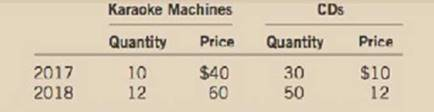# A small nation of ten people idolizes the TV show The Voice. All they produce and consume are karaoke machines and CDs, in the following amounts: a. Using a method similar to the CPI, compute the percentage change in the overall price level. Use 2017 as the base year and fix the basket at 1 karaoke machine and 3 CDs. b. Using a method similar to the GUP deflator, compute the percentage change in the overall price level. Also use 2017 as the base year. c. Is the inflation rate in 2018 the same using the two methods? Explain why or why not.### Principles of Macroeconomics (Mind...

7th Edition
N. Gregory Mankiw
Publisher: Cengage Learning
ISBN: 9781285165912

#### Solutions

Chapter
Section### Principles of Macroeconomics (Mind...

7th Edition
N. Gregory Mankiw
Publisher: Cengage Learning
ISBN: 9781285165912
Chapter 11, Problem 5PA
Textbook Problem
60 views

## A small nation of ten people idolizes the TV show The Voice. All they produce and consume are karaoke machines and CDs, in the following amounts:a. Using a method similar to the CPI, compute the percentage change in the overall price level. Use 2017 as the base year and fix the basket at 1 karaoke machine and 3 CDs.b. Using a method similar to the GUP deflator, compute the percentage change in the overall price level. Also use 2017 as the base year.c. Is the inflation rate in 2018 the same using the two methods? Explain why or why not.

Subpart (a):

To determine
Measuring percentage change in price using CPI and GDP deflator.

### Explanation of Solution

Cost of market for the basket can be calculated by using the following formula.

Substitute the respective values in Equation (2) to calculate the cost of market basket in the year 2017:

Cost of market basket for the year 2017 is $70. Substitute the respective values in Equation (2) to calculate the cost of market basket in the year 2018: Cost of the market basket2018=1×60+3×12=96 Cost of market basket for the year 2018 is$96

Subpart (b):

To determine
Measuring percentage change in price using CPI and GDP deflator.

Subpart (c):

To determine
Measuring percentage change in price using CPI and GDP deflator.

### Still sussing out bartleby?

Check out a sample textbook solution.

See a sample solution

#### The Solution to Your Study Problems

Bartleby provides explanations to thousands of textbook problems written by our experts, many with advanced degrees!

Get Started

Find more solutions based on key concepts
(Oligopoly Power) What are three sources of oligopolies?

ECON: MICRO4 (New, Engaging Titles from 4LTR Press)

Define depreciation as it relates to a van you bought for your business.

College Accounting (Book Only): A Career Approach

OPTIMAL CAPITAL BUDGET Hampton Manufacturing estimates that its WACC is 125%. The company is considering the fo...

Fundamentals of Financial Management, Concise Edition (with Thomson ONE - Business School Edition, 1 term (6 months) Printed Access Card) (MindTap Course List)